# Address Function in Microsoft Excel

To return the cell reference as text, we will use the Address Function in Microsoft Excel.

ADDRESS:- This function is used to creates a cell reference as text, given specified row and column numbers.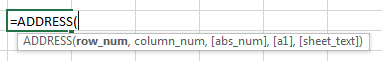There are 4 options for abs_num:-

a) Absolute cell Reference (1) :- Address returns an absolute cell reference.

b) Absolute row/ Relative column (2) :- Address returns an absolute row with a relative column.

c) Relative row/ Absolute column (3) :- Address returns a relative row with an absolute column.

d) Relative (4) :- Address returns a relative cell reference.

For example:

• Select the cell A1, and write the formula.
• The function will return the cell address \$A\$2.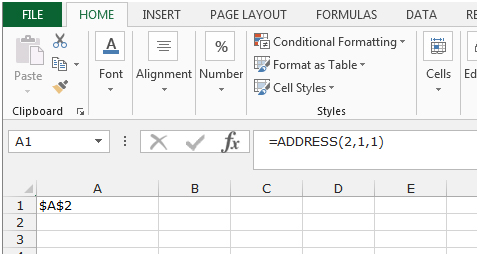We can use the ADDRESS function in 5 different ways.

Syntax Description
\$C\$2 Absolute reference
C\$2 Absolute row; relative column
R2C Absolute row; relative column in R1C1 reference style
[Book1]Sheet1!R2C3 Absolute reference to another workbook and worksheet
'EXCEL SHEET'!R2C3 Absolute reference to another worksheet

Firstly, lets understand all the above ways of using the Address function.

Absolute Reference

The absolute reference is used where the column and row references are fixed.

• Write the Address function for absolute reference in any cell.
• =ADDRESS(2,3), press Enter on the keyboard.
• The function will return\$C\$2.
• In this formula,2 is used to denote the 2nd row and 3 is used to denote the 3rd column, hence we get \$C\$2.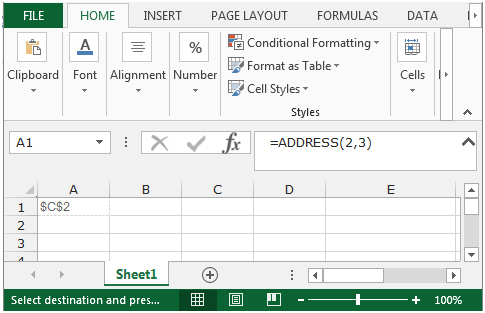Absolute row; Relative column

The absolute row and relative column referencesare used, where only the row reference s fixed.

• Write the Address function for Absolute row and Relative column in any cell
• =ADDRESS(2,3,2), press Enter on the keyboard.
• The function will return C\$2.
• In this formula, 2 is used to denote the 2nd row, 3 is used to denote the 3rd column and the 2 is used to denote the absolute row and relative column format for this reference.  Hence, we get C\$2.
• Note: As shown earlier, you can choose 1 among the 4 options for the abs_num parameter.  Hence if you use this formula =ADDRESS(2,3,3) it will return \$C2 where the column reference is fixed and the row is relative.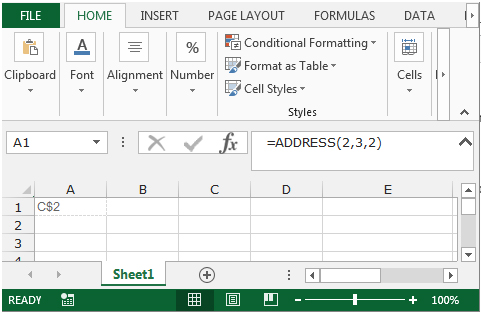Absolute row; relative column in R1C1 reference style

The absolute row and relative column references are used and the cell references are shown in the R1C1 format.

• Write the Address function for Absolute row and Relative columnin R1C1 reference style.
• =ADDRESS(2,3,2,FALSE), press Enter on the keyboard.
• The function will returnR2C.
• In this formula, 2 denotes the row, 3 denotes the column, 2 is to show the absolute row and relative column format for the reference.  And the FALSE is to show the cell reference in R1C1 style.  In place of False / True, you can also use 0 /1, where 0 is for R1C1 style, 1 is for A1 style. Hence we get the result as R2C.  So starting from the 1st cell and 1st column in the sheet, then R denotes ROW, 2 means 2nd row, C is for COLUMN and  denotes 3 columns to the right, which is column C.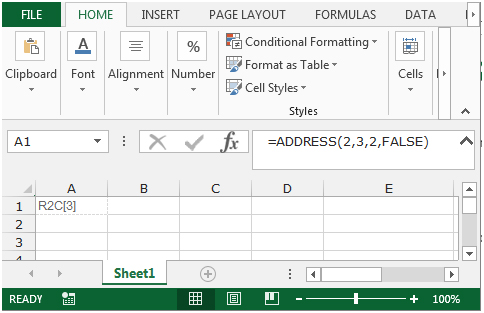Absolute reference to another workbook and worksheet

To show the absolute reference to another workbook and worksheet, follow the below given steps:-

• Write the Address function to show the Absolute reference to another workbook and worksheet.
• =ADDRESS(2,3,1,FALSE,"[Book1]Sheet1"), Press Enter on the keyboard.
• The function will return [Book1]Sheet1!R2C3.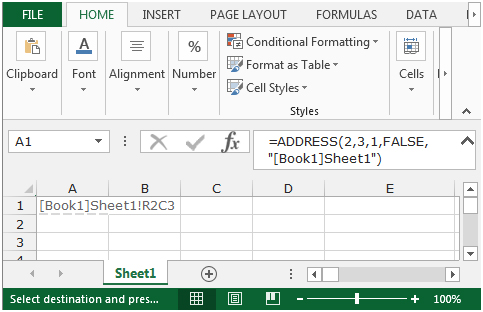In this formula,the only difference is the last parameter where we get the cell references with the name of the workbook and worksheet.  All other parameters are the same as explained above.

Absolute reference to another worksheet

To show the absolute reference to another worksheet, follow the below given steps:-

• Write the Address function to show the Absolute reference to another workbook and worksheet.
• =ADDRESS(2,3,1,FALSE,"EXCEL SHEET"), Press Enter on the keyboard.
• The function will return'EXCEL SHEET'!R2C3.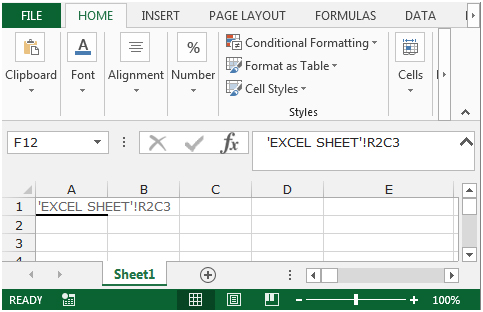In this formula, we get the absolute cell reference with the name of the worksheet which is “EXCEL SHEET”.

These are the ways to get the Cell Reference as Text in Microsoft Excel.We would love to hear from you, do let us know how we can improve, complement or innovate our work and make it better for you. Write us at info@exceltip.com

1.I am trying to do this with a defined area in an array and INDIRECT does not work. Any ideas?

2."This formula will return the contents of the cell:
3.